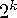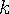The MEANS Procedure

# TYPES Statement

Identifies which of the possible combinations of class variables to generate.
 Requirement: CLASS statement Main discussion: Output Data Set Featured in:

 TYPES request(s);

request(s)

specifies which of thecombinations of class variables PROC MEANS uses to create the types, whereis the number of class variables. A request is composed of one class variable name, several class variable names separated by asterisks, or ().

To request class variable combinations quickly, use a grouping syntax by placing parentheses around several variables and joining other variables or variable combinations. For example, the following statements illustrate grouping syntax:

 Request Equivalent to types A*(B C); types A*B A*C; types (A B)*(C D); types A*C A*D B*C B*D; types (A B C)*D; types A*D B*D C*D;

 Interaction The CLASSDATA= option places constraints on the NWAY type. PROC MEANS generates all other types as if derived from the resulting NWAY type. Tip: Use ( ) to request the overall total (_TYPE_=0). Tip: If you do not need all types in the output data set, then use the TYPES statement to specify particular subtypes rather than applying a WHERE clause to the data set. Doing so saves time and computer memory.

The analyses are written to the output in order of increasing values of the _TYPE_ variable, which is calculated by PROC MEANS. The _TYPE_ variable has a unique value for each combination of class variables; the values are determined by how you specify the CLASS statement, not the TYPES statement. Therefore, if you specify

```class A B C;
types (A B)*C;```

then the B*C analysis (_TYPE_=3) is written first, followed by the A*C analysis (_TYPE_=5). However, if you specify

```class B A C;
types (A B)*C;```

then the A*C analysis comes first.

The _TYPE_ variable is calculated even if no output data set is requested. For more information about the _TYPE_ variable, see Output Data Set.Previous Page | Next Page | Top of Page